﻿ Evolution of the CAPM: From “Premium for Risk” to “Sharp-Linter-Black Model”Publications are Open
Access in this journal
Article Versions
Export Article
• Normal Style
• MLA Style
• APA Style
• Chicago Style
Review Article
Open Access Peer-reviewed

### Evolution of the CAPM: From “Premium for Risk” to “Sharp-Linter-Black Model”

Huseyin Yilmaz
Journal of Finance and Accounting. 2022, 10(1), 1-6. DOI: 10.12691/jfa-10-1-1
Received January 01, 2022; Revised January 29, 2022; Accepted February 07, 2022

### Abstract

The evolution of the Capital Assets Pricing Model (CAPM) started with the Williams  with the formula of “Premium for Risk”. Then, Hicks  and Markowitz  gave some opinions about risk premium and the value of an individual financial asset, respectively. Then, Treynor  and  brought some contributions to the model such as risk premium for equity and present price of a share. Sharp  added to the model the expected rate of return and he also transferred the standard deviation from statistics to the CAPM evolution. Linther  gave another risk premium approach with a different formula. Mossin  continued to improve the CAPM with his contributions of expected rate of return on a unit of a risky asset, return of a unit of a riskless asset, and the risk margin formulas. Black  completed the CAPM evolution with his model called “Sharp Linther Black Model”.

### 1. Introduction

Determination of values of assets has been an important subject in the past and today. There is no problem for government financial assets. Its value could be determined by discounting the principal and interest amount of a government bond or treasury bill. The discounting rate for government assets is called “risk free rate” or “riskless interest rate”.

The main issue for valuation of assets are about corporate financial assets. Its reason is risk exposure by the corporate assets. Investors are uncertain about corporate assets. Actually, the uncertainty levels of corporates are different from each other. There are two probabilities about corporations. The one of them is their being relatively successful, the other is their being relatively unsuccessful. For this reason, we should consider that every corporation has different degree of risk.

In this article, we will study an evolution of an asset pricing model which called CAPM (Capital Assets Pricing Model).

### 2. The Literature

Literature in this article has started considering the difference between market return and riskless interest rate (the risk Premium). Williams 1 has given a formula for risk premium while Hicks 2 has not defined any formula but he has tried to provide a definition for risk premium. Markowitz’s 3 contribution about the CAPM could be thought his omitting the first stage of his portfolio selection model. The omitting could be thought a reason or an opportunity for the writers in the theory of finance to remember the valuation of individual financial assets in the portfolio. Treynor 5 has determined a formula to calculate the risk premium together with his valuable contributions to the CAPM theory. Then, Sharp 6 improved a method to calculate “expected rate of return of combined assets (ERc)”. He also added his analysis standard deviation of return of combined assets (σRc). Linther 7 gave a formula for risk premium, too. Mossin 8 determined expected return of risky assets, expected return of riskless assets, and risk margin. Black 9 generalizes the “Required (Expected) Return of Return (RRR) formula called by the Fama &French 10 as “Sharp-Linther-Black” model.

In this article, the two books and the eight articles from William 1 to Black 9 have been accepted as the stages of the CAPM evolution. For this reason, they will be explained at the next section (the Section 3) in detail.

### 3. Stages of the CAPM Evolution

There are eight stages in the CAPM evolution. The stages are seen at the Table 1 below:

3.1. The First Stage (the Stage 1): Williams 

Williams 1 mentions uncertainty and the premium for risk at the” Chapter V “Evaluation by the Rule of Present Worth”. He points out that if an investor is uncertain about the future, it cannot tell for sure just what is the present worth of the dividends or of the interest and principal he will receive. He can only say that under one set of possible circumstances it will have one value and under another, another{1}. He adds to his opinions that a customary way to find the value of a risky security has always been to add a “premium for risk” to the pure interest rate, and then use the sum as the interest rate for discounting future receipt.

It could be seen from his opinions that he accepts risk premium and believes that it should be added to the pure interest rate{2} to discount future receipt.

He points out the mathematical definition of “premium for risk” like that:(1)

The symbols in the equation are defined as follows: x is the premium for risk; i is the yield at face value of interest and principal; and î is the pure interest rate.

His opinions have been a search a true discount rate to evaluate risky securities. The CAPM has same purpose, too. It aims to find the truest way to include risk to fix the value of a security. For this reason, Williams 1 could be accepted as the first stage in creating the CAPM.

3.2. Stage 2: Hicks 

Hicks 2 points out a concept “allowance for risk”. He defines the allowance for risk as the percentage by which the representative expected price falls short of or exceeds the most probable price, is not determined solely by the opinion of the planner about the degree of uncertainty. It is also influenced by his willingness to bear risks, by an element which in the last analysis depends upon his scale of preferences. Hicks thinks that for some purposes such as when an estimation is being made of the capital value of a person’s assets, it is sufficient to concentrate attention on the most probable value, and leave the rest of the frequency distribution out of account. However, for different purposes, the dispersion is very important. An increased dispersion would make an investor less willing to make plans which involve buying or selling on the date affected. If this is so, an increased dispersion will have the same effect as a reduction of the expected price, in cases where the individual plans to sell, as an increase of the expected price, in cases where he plans to buy. If the investor is to allow for uncertainty of expectations, we must not take the most probable price as the representative expected price, but the most probable price +/- an allowance for the uncertainty of the expectation, an allowance for risk.

He emphasizes the importance of risk premium because of uncertainty. The CAPM covers risk premium and uncertainty. The uncertainty is considered via variance in the denominator and covariance in the numerator in composing the Beta calculation. So, Hicks has contributed to the CAPM with these opinions. Hicks has not given any formula to explain the “allowance for risk” in Hicks 2. As a result, he accepts uncertainty, difference about risk preference among investors, sufficiency of the calculation of most probable value of assets, usefulness of dispersion to increase the price during selling and to decrease the price during buying. These are precursor of the CAPM. All of these concepts and opinions are considered during the calculation of required rate of return via the CAPM. For this reason, the Hicks 2 could be thought the second stage after Williams 1, the first stage.

3.3. Stage 3: Markowitz 

Markowitz 3 says that the process of selecting a portfolio may be divided into two stages. The first stage starts with observation and experience and ends with beliefs about the future performances of available securities. The second stage starts with the relevant beliefs about future performances and ends with the choice of portfolio. He says that his article is concerned with the second stage and he first considers the rule that the investor should maximize discounted expected returns. This rule is rejected both as a hypothesis to explain, and as a maxim{3} to guide investment behavior. After the rejection of return maximization, he explains his new rule. The new rule to consider is that the investor should consider expected return a desirable thing and variance of return an undesirable thing. He thinks that the new rule has many sound points, both as a maxim for, and hypothesis about investment behavior. He points outs that he used the new rule to explain his portfolio selection theory. He states that generally two types of rules are valid to calculate value of investment. One of them is that the investor should maximize the discounted value of future returns. The second and preferred rule by Markowitz is that the investor should consider expected return a desirable thing and variance of return an undesirable thing. I think, Markowitz has contributed the CAPM about the denominator of the Beta, the Variance.{4}

Markowitz says at the end of the paper that in his paper, he has considered the second stage in the process of selecting of a portfolio. This stage starts with the relevant beliefs about the securities involved and ends with the selection of a portfolio. He adds that he has not considered the first stage: the formation of the relevant beliefs on the basis on observation. I think making the relevant beliefs about the securities requires some scientific techniques. The CAPM was improved for this reason. Every security has different risk level and this should be reflexed portfolio return. This could be made very easily using the CAPM because it covers Beta and “risk premium{5}”. Actually, his portfolio theory has not been completed because of missing of the first stage. This situation or missingness actually shows the need to evaluate or calculate required rate of return being considered as candidate securities included to the portfolio. The required rate of return could be calculated via the CAPM. This need could be thought as a stage to start the CAPM theorem. That is, there is no formula for CAPM in Markowitz 3. However, the Markowitz shows a need for calculating the required rate of return frankly in the first stage. Markowitz discovers the need to determine required rate of return to use for the first stage. It could be the CAPM. For this reason, Markowitz 3 could be thought the third stage of the CAPM evolution.

3.4. Stage 4: Treynor [4,5]

Treynor 4 starts to think about the CAPM opinion with the discount rate used in the discounted cash flow method. He thinks that the discount rate should be calculated separately for companies to evaluate their investment. Then, he thinks that investment uncertainties are far from perfectly correlated in capital markets. It points out Beta implicitly because the systematic risks of different companies or securities are different. He says that for any class of investments, errors in the performance of which are perfectly correlated, the present market value or price will tend to be equal to the linear function of expected value and uncertainty. He also thinks that correlation coefficient of the companies or securities in same industry is +1. That is, the securities change in same route and in same rate. Treynor discusses about risk premium. He thinks that the future value v may be sensitive to other economic variables for which there is a substantial risk premium. He adds that if a future value is certain or dependent only on insurable economic variables, then the market should tend to discount it at the risk-free rate of interest. He adds to his opinions that one can substitute for future market value as a function of the relevant economic variables the expected future value, with respect to the insurable variables, as a function of the uninsurable variables, since the contribution of insurable variables to the risk premium is, by definition, negligible. The resulting function is dependent only on the uninsurable variables, projections of which are, by assumption, continuous functions of time. He says a projected future value covers an expected value with respect to any relevant insurable economic variables and a function of market projections of any relevant uninsurable variables.

As it could be seen, he points out expected value, insurance, correlation, performance errors, future value, insurable economic values, and systematic risk and the Beta. He implies that systematic risks of investments are different than each other. These concepts mean risk and uncertainty. He exactly decides that an investor is under risk and uncertainty and he/she/it (investor company) needs to manage his investments carefully.

Treynor 5 highlights that the effects of risk on shifts in the market consensus occur over time, rather than on differences among investors at a point in time. He says that his own investor style diversifies to cope with equity risk, not except interest rate risk. Equity risks are so large that as if cash and bonds seem almost riskless. He thinks required return for a risky investment covers a risk-free rate and an expected return of risk taking. Treynor says that the risk premium is an important concept introduced by this paper. Variances and covariances are used to characterize the uncertainty in the yield of shares. He adds to his opinions that apparently it is a mistake to expect the risk premium to depend only on the sheer magnitude of the risk. If the uncertainty in stock is small, or the uncertainty is not small but orthogonal to the market as a whole, then the risk premium will be small. The latter possibility would result in a small risk premium even for a large risk. However, the appraisal problem is not trivial for uninsurable risks. Treynor gives a formula to calculate “risk premium” for an equity. It could be shown like that:(2)

The symbols in the equation are defined as follows: ai is the risk premium for the (i) th equity; b is the one period discount factor defined in terms of the lending rate r by b=1/(1+r); vi (1) is the future value of the (i) th equity; vi (0) is the present (certain) price of a share in the (i) th equity; and vi(1) is the expected value of vi (1).

From this formula, we can reach to vi(0), present (certain) price of a share in the (i) th equity. It could be derived like that: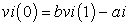(3)

The same meanings of the letters used in the formula 2 is valid for vi(0) formula, the formula 3. So, Treynor considers (adds) the risk premium to calculate present equity value.

Treynor 5 points out to the importance of equity investment, variances, covariances, and orthogonal uncertainty which brings small risk than that of equity risk. As it could be seen, he considers different level of risks. There is no “same level risk”. This brings out systematic risk and risk premium to be considered covered by the required rate of return. He approaches the CAPM opinion with his opinions and concepts he accepts. His risk premium insight is more detailed than that of th Williams 1 and Hicks 2. For this reason, the Treynor 4 and 5 could be thought the fourth stage of the CAPM evolution.

3.5. Stage 5: Sharp 

Sharp 6 points out that after preparing this paper he learned that Treynor had independently developed a model similar in many respects to the one described here, but it was unpublished yet to discuss here. Sharp thinks that riskless asset’s, P, risk is zero that’s σRp=0 and its rate of expected return, ERp, is equal by definition to the pure interest rate {6}. If an investor places α of his wealth in P and the remainder in some risky asset A, his expected rate of return could be calculated as below: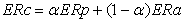(4)

The symbols in the equation are defined as follows: ERc is the expected rate of return of combined assets (risky plus riskless assets); α is the riskless asset; ERp is the expected pure (riskless) interest rate; and ERa is the expected rate of risky asset. The standard deviation of such a combination would be: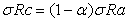(5)

The symbols in the equation are defined as follows: α is the riskless asset; σRc is the standard deviation of return of combined assets; and σRa is the standard deviation of return of risky assets.

Sharp accepts two assumptions in order to derive conditions for equilibrium in the capital market. These are a common pure rate of interest with all investors able to borrow or lend funds on equal terms, and homogeneity of investor expectations. That is, investors are assumed to agree on the prospects of various investments such as the expected values, standard deviations, and correlation coefficients. He accepts in advance that these assumptions are highly restrictive and undoubtedly unrealistic. However, he asserts the assumptions that since the proper test of a theory is not the realism of its assumptions but the acceptability of its implications, and since these assumptions imply equilibrium conditions which form a major part of classical financial doctrine, it is far from clear that this formulation should be rejected especially in view of the dearth of alternative models leading to similar results. He thinks that it is important to recognize that many alternative combinations of risky assets are efficient and thus the theory does not imply that all investors will hold the same combination. On the other hand, all such combinations must be perfectly (positively) correlated, since they lie along a linear border of the ER and σR region. This provides a key to the relationship between the prices of capital assets and different types of risk. However, there is no linear relationship between for individual assets. Typically, the ER and σR values associated with single assets will lie above the capital market line, reflecting the inefficiency of undiversified holdings. Moreover, such points may be scattered throughout the feasible region, with no consistent relationship between their expected return and total risk (σR). However, there will be a consistent relationship between their expected returns and systematic risk. He terms an asset’s total risk as the systematic risk. The part of an asset’s risk which is due to its correlation with the return on a combination cannot be diversified away when the asset is added to the combination. Diversification enables an investor to escape all risks except the risk resulting from swings in economic activity. This type of risk remains even in efficient combinations. Since all other type risks can be avoided by diversification, only the responsiveness of an asset’s rate of return to the level of economic activity is relevant in assessing its risk. Prices will adjust until there is a linear relationship between the magnitude of such responsiveness and expected return. Assets which are unaffected by changes in economic activity will return the pure interest rate. Assets which move with economic activity will promise appropriately higher expected rates of return. His opinion is that there is a consistent relationship between systematic risk and expected return. This relationship is not valid for total risk.

3.6. Stage 6: Linther [7,11]

Linther 7 says that “Sharp’s paper 6 appeared after this paper was in final form and on its way to the printers. He says that his first section which parallels the first half of Sharp’s paper (with corresponding conclusions), sets the algebraic framework for sections II,III, and VI, (which have no counterpart in Sharp’s paper) and for section IV on the equilibrium prices of risk assets, concerning which his results differ significantly for reasons which will be explored elsewhere. He adds that Sharp does not take up the capital budgeting problem developed in section V.

Linter points out that the result of the paper is not being presented as directly applicable to practical decisions, because many of the factors which matter very significantly in practice have had to be ignored or assumed away. The function of these simplifying assumptions has been to permit a rigorous development of theoretical relationships and theorems which reorient much current theory and provide a basis for further work. Linther thinks that before finding the optimal stock mix, it is necessary to express the return on any arbitrary mix in terms of the returns on individual stocks included in the portfolio. {7}

Linther calculates risk premium like that:(6)

The symbols in the equation are defined as follows:is the risk premium;is the market interest rate; and r* is the riskless interest rate.

He points out that for risky securities (i.e., those with standard deviation i and square of standard deviation i > o) to be held long by optimizing risk-averse investors in perfect markets need not always be positive as generally presumed. He says that since the covariances between most pairs of stocks will be positive, stocks held long in a portfolio will generally be those whose expected return is enough greater than the risk-free rate to offset the disutility of the contribution of their variance of pooled covariance to the risk of the entire portfolio. He adds that this is a standard doctrine. In addition to positive risk premium risky securities, the stocks whose expected returns are less than the riskless rate will also be held long provided that either they are negatively correlated in sufficient degree with other important stocks held long in the portfolio or that they are positively correlated in sufficient degree with other important stocks held short in the portfolio. This decreases variance of portfolio and stock itself. This is valid for securities with positive correlations held short in the portfolio, too. He adds his opinion that long position for positive risk premium and short position for negative risk premium are not necessary. Linther thinks that it could be concluded that risk premiums could most simply and plausibly be related directly to variances of returns. He also thinks that since the principal function of the concept of “risk class” has been to delineate a required level of risk premium, it could be concluded further that risk classes should also be delineated in the same units (variances) if the concept of “risk class” should be used at all.

Linther 11 thinks that the total risk on a given security is the sum of the variance of its own dollar return over the holding period and the combined covariance of its return with that of all other securities. This total risk on each security is priced up by multiplying by a market price of dollar risk which is common to all securities in the market. The expected dollar returns on any security less this adjustment for its risk gives its certainty-equivalent dollar return, and the market price of each security is simply the capital value of this certainty-equivalent return using the risk-free interest rate. He determines price including risk with expected return on security and certainty equivalent price of an assets using riskless interest rate. It shows his sensitivity on risk. He is so sensitive to risk that he separates the interest rate (discount rate) used to calculate the expected value.

3.7. Stage 7: Mossin 

Mossin 8 says that Sharp 6 gives a verbal-diagrammatical discussion of the determination of asset prices in quasi-dynamic terms. He thinks that Sharp’s general description of the character of the market is similar to the one presented here, and Sharp’s main conclusions are certainly consistent with his. But Sharp’s lack of precision in the specification of equilibrium conditions leaves parts of his arguments somewhat indefinite. Mossin 8 says that Mossin 8 may be seen as an attempt to clarify and make precise some of these points. Mossin says that the portfolio analysis mentioned earlier assume that, in his choice among all the possible portfolios, the individual is possible to be guided by its expected yield and its variance only. He says that the assumption will also be made in this paper. He accepts the concept “value” that to be considered on any asset on a given market date may than be thought of as the value per unit that the asset will have at the next market date (including possible accrued dividends, interest, or other emoluments). The terms “yield” and “future value” may then be used more or less interchangeably. Mossin says that the expected rate of return rj on a unit of a risky asset can be defined by(7)

The symbols in the equation are defined as follows: rj is the expected rate of return of j; µj is the probability of gaining of the return of j (in the uncertainty conditions); and pj is the price per unit of asset j.

Then, Mossin continues his explanation that the rate of return of a unit of the riskless asset rn is defined by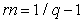(8)

The symbols in the equation are defined as follows: rn is the rate of return of a unit of the riskless asset and q is the current price.

Mossin thinks that in general, it could be thought of the rate of return of any asset as separated into two parts: the pure rate of interest representing the “price of waiting”, and a remainder, a risk margin, representing the “price of risk”. He says “when we set the future yield of the riskless asset at 1 and decided to fix its current price at q, we thereby implicitly fixed the pure rate of interest. And to say that the market determines only relative asset prices is seen to be equivalent to saying that the pure rate of interest is not determined in the market for risky assets. He thinks that the asset markets determine only the risk margins. The risk margin on asset j, mj, is defined by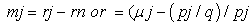(9)

The symbols in the equation are defined as follows: mj is the risk margin on asset j; rj is the expected rate of return of j; rn is the rate of return of a unit of the riskless asset; µj is the probability of gaining of the return of j (in the uncertainty conditions); pj is the price per unit of asset j; and q is the current price.

Mossin uses concept of “the price of risk reduction “and he defines it as “the amount of expected yield that must be sacrificed in order to reduce risk”. He adds that risk is measured by the value (in dollars) of the standard deviation of yield, then the dimension of the price of risk reduction is that of an interest rate.

He accepts the concept standard deviation, pure (riskless) interest rate, expected yield, price of risk, price of waiting for riskless interest rate, expected rate of return, future value etc. to explain his opinions.

3.8. The Last Stage (the Stage 8): Black 

Fama & French 10 says “Sharp Linter Black Model” for the CAPM. So, Black 9 could be considered a completing part of the CAPM.

Black 9 says that the CAPM states that under certain assumptions the expected return on any capital asset for a single period will satisfy: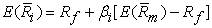(10)

The symbols in the equation are defined as follows:is the return on asset i for the period and is equal to the change in the price of the asset, plus any dividends, interest, or other distributions, divided by the price of the asset at the start of the period;is the return on the market portfolio of all assets taken together; Rf is the return on a riskless asset for the period; ßi is the “market sensitivity” of asset i and is equal to the slope of the regression line relatingand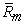. (Black (1972) says that the market sensitivity ßi of asset i is defined algebraically by:Actually, this formula is a completing part of the Formula 10. It shows calculation of the ßi in the formula10).

The evolution of the CAPM has been completed with this stage. The Sharp-Linther- Black model or formula has been being used in many textbooks, internet sources, and scientific researches today to calculate the “required (expected) rate of return” which is the output of the Sharp Linter Black model.

### 4. Conclusion

William 1 pointed out the uncertainty and risk premium. Hicks 2 explained risk premium, willingness to bear risks, scale of preferences, and probability.

Markowitz 3 showed the first stage of portfolio as value of the security which will be included to a portfolio. This points out a need for a capital asset pricing model.

Treynor 4 and 5 said that discount rates are different for different investments. Investment uncertainties were not perfectly correlated with capital markets, sensitivities of future value to risk premium were different, uninsurable variables were important for risk Premium, effects of risk on the shifts on market consensus were important, diversification to cope with equity risk and interest rate risk were valid. He thought about covariance and variance. I think it is a beginning point to the Beta calculation which was included in the Sharp-Linther-Black method.

Sharp 6 gave a formula covering risky and riskless assets. Sharp’s opinion covers expected values, standard deviation and correlation. Sharp thinks that there is a relationship between expected return and systematic risk.

Linther 7 and 11 accepted that standard deviation, square of standard deviation (variance), and covariance so he accepted the content of Beta. He thought that risk premium could be negative, too, negative risk premium stocks should have been being held long and positive risk premium stocks should have been being held short. He thought that, in so doing, the variance could be decreased.

Mossin 8 asserted that he clarified Sharp 6’s some unclear opinions. He formulated risk margin, expected rate of risky assets, and expected rate of riskless assets separately. He used the concept of the price of risk reduction and gave some details to determine the risk margin.

Black 9 determined the CAPM model which was called “Sharp-Linther-Black Model” by Fama &French 10.

As if, there was a consensus and a joint process to create the CAPM model among the theoreticians. It seems that, the opinions risk premium, standard deviation, variance, covariance etc. have been being added to each other by different economist and finance theoreticians. In my opinion, the opinions were pretty connected with each other and they completed each other, so the CAPM was created.

### Notes

1. I think that he means “under another set of possible circumstances, it will have another value”.

2. It could be thought as “riskless interest rate”. In my opinion, he does not consider inflation and so he does not consider “without inflation” with the term “pure”. It could be considered that his main idea in the chapter of the book is about the necessity of considering risk in discounting future returns.

3. It is written “maximum” in the Markowitz (1952:77). In my opinion, it should be written “maxim” logically because in the sentence the “maximum” is meaningless linguistically.

4. Beta =Covariance:Variance

5. Risk Premium = Market return- Riskless interest rate

6. Pure interest rate is interest rate on capital excluding payment for risk.

7. Ø= (r-r) / σr

### References

  Williams, J.B. (1938), The Theory of Investment Value, North Holland Publishing Company, Amsterdam, Netherland. In article  Hicks, J.R (1939), Value and Capital, Oxford University Press, Second Edition, Great Britain. In article  Markowitz H. (1952), “Portfolio Selecting”, The Journal of Finance, Vol.7, No.1, pp.77-91. In article View Article  Treynor J.L. (1961), “Market Value, Time, and Risk”, Unpublished manuscript, “Rough Draft” Dated 8.8.1961, 95-209, pp.1-45. In article View Article  Treynor J. L. (1962), “Toward A Theory of Market Value of Risky Assets”, Rough Draft, Revised 12/28/02, with minor edits by Craig William French, pp.1-19. In article  Sharp W. (1964), “Capital Asset Prices: A Theory of Market Equilibrium under Conditions of Risk”, The Journal of Finance, Vol. 19, No. 3, pp. 425-442. In article View Article  Linther J. (1965a), “The Valuation of Risk Assets and the Selection of Risky Investments in Stock Portfolios and Capital Budgets”, The Review of Economics and Statistics, Vol. 47, No.1, pp.13-37. In article View Article  Mossin J. (1966), “Equilibrium in a Capital Asset Market”, Econometrica, Vol. 34, No. 4, pp. 768-783. In article View Article  Black F. (1972), “Capital Market Equilibrium with Restricted Borrowing”, The Journal of Business”, Vol.45, No.3, pp.444-455. In article View Article  Fama E. and French R. (2004), “The Capital Asset Pricing Model: Theory and Evidence”, Journal of Economic Perspectives, Vol.18, No.3, pp.25-46. In article View Article  Linther J. (1965b), “Security Prices, Risk and Maximal Gains From Diversification”, The Journal of Finance, Vol.20, No.4, pp.587-615. In article View ArticleThis work is licensed under a Creative Commons Attribution 4.0 International License. To view a copy of this license, visit http://creativecommons.org/licenses/by/4.0/